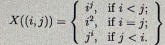Apr 25, 2023

Part 1:

Question 1. Prove by Mathematical Induction, or disprove, that a! ≤ aa, for all natural numbers a ≥ 2.

Question 2. Prove by Mathematical Induction, or disprove, that any natural number j can be written as a sum of non-negative power(s) of 2.

Question 3 (a) 63 ∈ S.
If x ∈ S, then x + 7 ∈ S.
Based on the above recursive definition, describe S in at most two sentences.

(b) λ ∈ T.

If y ∈ T`, then "1" y "0" ∈ T and "1" y"1" ∈ T.
Write down any five non-empty strings in T`.
Use at most two sentences to describe the elements of T.

Question 4. Consider the poset ({2, 3, 4, 6, 8, 12, 15, 20, 24, 30}, |).
(a) Write down all the maximal element(s). (Write down `Nil` if there isn`t any maximal element.)
(b) Write down all the minimal element(s). (Write down `Nil` if there isn`t any minimal element.)
(c) Write down all the upper bound(s) of {4, 6}. (Write down `Nil` if there isn`t any upper bound.)
(d) Write down all the lower bound(s) of {12, 24}. (Write down `Nil` if there isn`t any lower bound.)

Question 5. Two standard decks are mixed and used in a card game.
(a) How many cards do we need to draw to guarantee that ten cards are having the same color? (Steps required.)
(b) How many cards do we need to draw to guarantee that we have three red cards in the range of 4 to 7 (inclusive)? (Steps required.)

Question 6. P = {a, b, c, d, e, f}, Q = {a, e, i, o, u]. How many onto functions can be formed from P to P - Q? (Steps required.)

Question 7. A pair of fair dice is rolled. Let X((i, j)) be the random variable, where i and j are the numbers that appear on the first die and the second die, respectively.

X((i, j)) is defined as follows:(a) What is the distribution of the random variable X? (Steps required.)
(b) What is the expected value X? Correct the answer to 4 decimal places. (Steps required.)
(c) What is the variance of X? Correct the answer to 4 decimal places. (Steps required.)

Part 2:

Question 1

If the statement is true, prove it by Mathematical InduQion.
If the statement is false, give a counterexample.

Question 2.
If the statement is true, prove it by Mathematical Induction. If the statement is false, give a counterexample:

Question 3. (a) Write AT MOST TWO statements to describe the set S.

(b) Write down five elements ofT.

Write AT MOST TWO statement to describe the elements of the set T.

Question 4. You may draw the Hasse diagram on a draR paper and obtain the answers.

Question 5. You should consider whether the Pigeonhole Principle can be applied.

Question 6. This is a counting problem.

Question 7. (a) For each possible outcome, the X value should be computed. For each possible X value, the probability should be computed.

(b) The expected value of X should be computed.

(c) The variance of X should be computed.